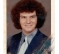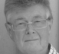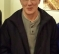## ChatGPT's idea of a computationally efficient digital filter - ha ha ha...Started by 8 months ago18 replieslatest reply 7 months ago398 views

Design a computationally efficient digital filter which can extract a 1 kHz signal between 1000 Hz and 2000 Hz from a digital input signal with a sample rate of 100 kHz.

A computationally efficient digital filter that can extract a 1 kHz signal between 1000 Hz and 2000 Hz from a digital input signal with a sample rate of 100 kHz can be designed using a finite impulse response (FIR) filter. The design of an FIR filter involves specifying the desired frequency response, which in this case would be a bandpass filter with a passband between 1000 Hz and 2000 Hz, and a transition bandwidth that is narrow enough to effectively remove any signals outside of the passband. The desired frequency response can then be transformed into a set of filter coefficients using techniques such as the window method.

Here is a simple design for an FIR bandpass filter using a rectangular window:

1. Specify the filter length, N, and the passband frequency range, fc1, fc2. For this example, we will use N = 31 and fc1 = 1000 Hz, fc2 = 2000 Hz.
2. Calculate the transition bandwidth, Δf, and the cutoff frequencies, f1, and f2. The transition bandwidth is equal to fc2 - fc1, and the cutoff frequencies are equal to fc1 - Δf/2 and fc2 + Δf/2.
3. Generate the impulse response, h[n], of the filter using the rectangular window method. The impulse response is given by:

h[n] = 2Δf * sin(2πfc1n/fs) / (2πfc1n/fs) for 0 <= n <= (N-1)/2 h[n] = 2Δf * sin(2πfc2n/fs) / (2πfc2n/fs) for (N-1)/2 < n <= N-1

1. Normalize the impulse response so that the gain of the filter at the center frequency of the passband is equal to 1.

The resulting impulse response, h[n], represents the filter coefficients that can be used to extract the desired 1 kHz signal from the input signal. The input signal can be filtered by convolving it with the impulse response, h[n], to obtain the filtered output signal. This can be done efficiently using fast convolution algorithms such as the fast Fourier transform (FFT).

Note: This is a simple example of designing an FIR bandpass filter, and more advanced methods such as using a Kaiser window or an optimization algorithm can be used to design a filter with improved performance.

[ - ]Why not use an IIR filter? Ptc.Controls.Worksheet.Printing.pdf

[ - ]Hi Kenneth,

That was my first reaction, too. It seems like that would be an obvious choice.

--Randy

[ - ]The IIR filter is even worse as an ill conditioned problem. Anytime there is a large ratio of sample rate to BW (100 to 1 here) the passband poles are packed very close together even when they are unpacked in biquadratic 2-nd order filters. The poles repel each other (move away from their center of gravity) due to finite arithmetic errors in proportion to the reciprocal of the distance between them (sensitivity analysis) see attached paper

[ - ]fred,

Thanks for that paper! I was reviewing an older forum post on this problem which mentioned Rick's "DSP Tips and Tricks" paper in the March 2009 issue of IEEE Signal Processing Magazine, which I promptly retrieved, and noticed what looks like your Figure 4 in that article and was wondering how you determine those points. More reading to be done...

--Randy

[ - ]A good rule to follow in DSP design is don't build filters when there is a large ratio of sample rate to BW. The response to this advice is to reduce the sample rate with a down sampling signal conditioning filter.

I gave some thought to this problem, sample rate 100 kHz, bandwidth, a span between 1 kHz and 2 kHz. transition BW of filter in this span is 0.5 kHz. I selected 80 dB stop band attenuation. The brute force filter length is approximately

(fs/trans BW)*attn(dB)/22 = (100/0.5)*(80/22), approx 720 taps. This runs at 100 kHz input clock sample rate.

Let's do this instead: Use a 10 path filter to reduce sample rate
10-to-1 from 100 kHz to 10 kHz. Passband BW is 0 to 2 kHz, folding frequency is 5 kHz, transition BW from 2.5 kHz to 7.5 kHz, This first filter has a length (100/5)(80/22), approximately 72 taps in ten paths, we can round up to 80 taps or 8 taps per path which is 8 ops per input sample. we now have the alias free interval in 1-to-2 kHz sampled at 10 kHz.

We have a few options now.

we can design the bandpass filter at the reduced sample rate which is now 72 taps running at 10 kHz. This adds 7.2 ops relative to the input rate for a total less than 16 ops per input sample.

Alternatively, the second filter can be embedded in a ten path filter which performs a 5-to-1 down sample from 10-kHz to 2-kHz. This filter uses about 16 ops per input sample but when referred to input rate, is 1.6 ops per input for a workload less than 10 ops per input with output rate of 2-kHz. We can follow this second down sampling filter with a 1-to-5 or a 1-to-8 upsampling filter which would raise the workload to less that 12 ops per input sample.

I'll write the scripts to implement one or two of the options and post them in a bit.

The 10 path filter allows us to form the bandpass filter between 1-kHz and 2-kHz with DC being a boundary between adjacent filter bands instead of DC being a center of the 0 indexed bin (even centered or odd centered channels) This is true for a full channelizer and must also be true for a single channel. the 1-to-2 kHz band is centered at 1.5 kHz sampled at 10 kHz. With the 5-to-1 down sample in the second 10-path filter, the 1.5 kHz aliases to the -quarter sample rate (1.5/10)*(5)= 1.5/2. This residual spinning is removed with trivial de-spinning by multiple of j^n in a 4-state state machine. You can see this in my chapter on even-odd centered channelizers in my second edition of multirate DSP.

More later

fred harris

[ - ]here is my script for the two stage polyphase filter. two versions

version 1 a 10 path polyphase filter that performs a 10-to-1 down sampler from 100 kHz to 10 kHz. This is followed by a single bandpass filter that extracts the band in the spectral span 1 kHz to 2 kHz without changing sample rate.

then the second option uses the same second filter in a 10-path polyphase filter to perform a 5-to-1 resample from 10 kHz to 2 kHz. It aliases the band centered at 1.5 kHz (spanning 1 kHz to 2 kHz) to baseband at the 2-kHz sample rate. This in turn is followed by a 1-to-5 up sampling interpolator that returns the sample rate to 10 kHz and then heterodynes the baseband signal centered at 0 back to 1.5 kHz. I got lazy here. I should have used the dual of the 5-to-1 down sample to up sample and translate simultaneously. I will do that later

I will write a short paper soon explaining all the steps. the myfrf is called by the modified remez algorithm. (better interpolator with 1/f stop band sidelobes.) put them both in the same directory

Have fun playing... This is a very efficient way to extract narrow band signals embedded in a high sample rate data set. The workload drops from 800 or so operations at 100 kHz to some 10 operations or so... order of magnitude work load reductions are common.

I'm always open for questions.

fred

Single_channel_100.m

myfrf.m

[ - ][ - ]We need more constraints. Is the signal actually 1 kHz or is it an unknown frequency signal in the interval 1.0 to 2.0 kHz band? Is it a real signal or is it a complex signal? Is there any noise added to the sinusoid? What is the nominal signal level and what is the nominal noise level? What is the number of samples of the noisy sinusoidal sequence. Do you want the actual samples of the extracted sinusoid and do you want to maintain the 100 kHz sample rate? Can we reduce the sample rate? How many bits are there in the quantized sampled data? When we have the full statement of the problem... the answer will follow. Good interview problem.

fred h

[ - ]Hi Randy.

When I model the following robot equations:

"h[n] = 2Δf * sin(2πfc1n/fs) / (2πfc1n/fs) for 0 <= n <= (N-1)/2 h[n] = 2Δf * sin(2πfc2n/fs) / (2πfc2n/fs) for (N-1)/2 < n <= N-1"

I arrive at an h[n] that is not even close to a sensible bandpass filter impulse response. "Forbidden Planet's" Robie the Robot would be embarrassed by the ChapGPT robot.[ - ]Hi Rick,

Interesting experiment! I did not think to actually check out that impulse response. Robie may have a vacuum tube (or two) out..

--Randy

[ - ]Hi fred,

These are all excellent questions on the parameters of the problem which of course you'd have to answer in a real-world scenario. Are you implying that, had I specified these parameters, ChatGPT would have presented a more appropriate solution?

-Randy

PS: It is an honor to "meet" you, even virtually! I've admired you as a signal processing engineer since the 80s (as have thousands (?) of others) when I first came across your paper "On the use of windows...".

[ - ]Thanks for the nice feedback randy.

I tell my students that my housekeeper doesn't do windows, but I do!

have you played with the latest matlab script i put on line for the efficient filter?

fred

[ - ]Post deleted by author

[ - ]Hmm it can write a great poem about the Nyquist theorem (all frequencies so nice, they must be sampled twice) but seems to have slightly harder time with actual application

[ - ]Hi jbrower,

Exactly! I'd seen several instances (including Rick's recent forum post) about some artistic endeavor such as writing lyrics, but I wanted to present something which required real thought as a test.

-Randy

[ - ]Randy and Fred

I have seen theese papers telling me that it is difficult to make an IIR-filter with a high sampling to bandwidth ratio. This is so for the poles are close to 1 in the Z diagram. However, as the coefficients becomes close to 2 and 1 it is possible to change the circuit and with just 3 extra additions it is possible to get close to 64 bits precision by using 32 bit multiplications and additions with a 24 bit datastream from an A/D converter. For further information: https://iirfilter.webnode.se/

[ - ]Hello all,

Here it is: a tone signal in the spectral interval 1 kHz to 2 kHz. sampled at 100 kHz, Filter to extract tone, Passband 1-kHz to 2-kHz, stop band 0 to 0.5 kHz and 2.5 kHz to 50 kHz. Transition BWs is 0.5 kHz. 80 dB stop band attenuation. The filter that can do this contains approximately 800 taps and operates at 100 kHz. Don't do this. Instead build a 10-path polyphase channelizer that performs a 10-to-1 down sample with passband 0 to 2.5 kHz and with stopband 5 kHz to 50 kHz. this filter has 80 taps and requires 8 operations per input point at 100 kHz sample rate. The desired 1-kHz to 2-kHz band is contained in the low pass bandwidth. We now can address the band pass filter operating at the reduced 10 kHz sample rate. This filter now only has 80 taps (one tenth due to 10-to-1 down sample) in it and is operating at one-tenth the clock rate for a work load reduction of 99%, less than 1-operation per input sample referred to the input sample rate. But we can do more. we can use the second filter as another 10-path filter and perform a 5-to-1 resample from 10 kHz to 2-KHz. This again reduces the workload per input sample (second filter) to 16 ops per input (instead of 80 at the reduced 10 kHz sample rate, another 5-to-1 workload reduction). The desired signal band is aliased to DC and operates at 2 kHz sample rate. We then build its dual graph, synthesis filter, a 10 path filter that up samples the 2-kHz to 10-kHz and aliases the DC band back to the 1-kHz to 2-kHz spectral span. This workload is the same as the 5-to-1 down sample.. the entire workload is less than 10 ops per input sample. Slightly better than the 800 ops direct brute force approach. If we want the 100 kHz sample rate we could build the Dual of the input 10-to-1 down sample as a 1-to-10 up sample and double the workload to 20 ops per input-output process. Run the attached script. I'll write a short paper for an up-coming conference.

It was fun

fred h

myfrf.m

Single_channel_100_2.m

[ - ]